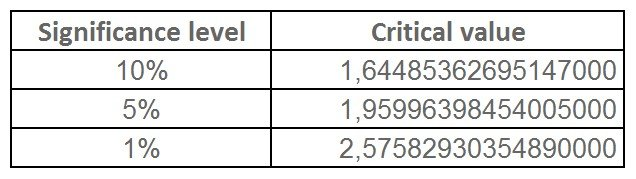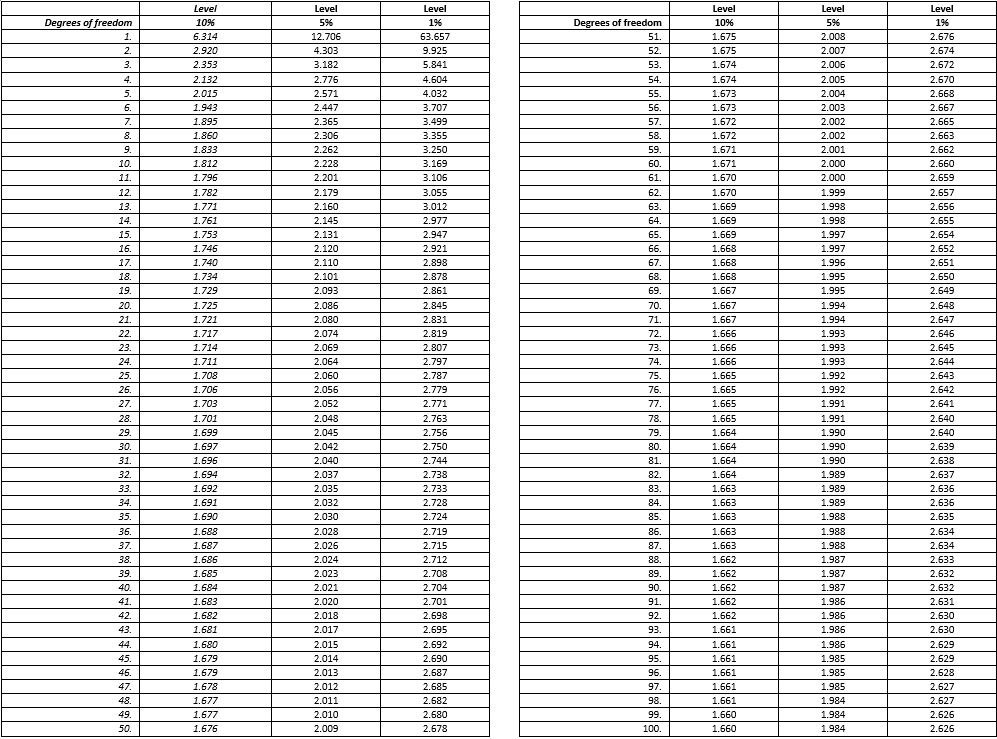The following input values are used in the formula:

p = norm value
x= test value (a % value)
n= base size* of x
q=100-p

Note: The base size is weighted if the test value (x) is weighted.

Formula:

Z=diff/se
odiff= ABS
ose= √ (p x q / n)

Critical Values, n >100

The following critical values are used when the base size of the tested value is above 100.Critical Values, n <=100
The list below shows the critical values used when the base size is below 100.

*Degrees of freedom = n (the base size of the test value)